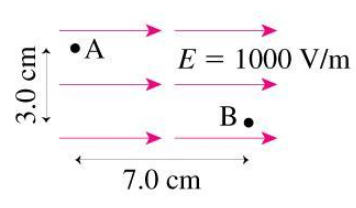# Problem: What is the potential difference between A and B?

###### FREE Expert Solution

Electric field:

$\overline{){\mathbf{E}}{\mathbf{=}}{\mathbf{-}}\frac{\mathbf{∆}\mathbf{V}}{\mathbf{∆}\mathbf{x}}}$

The electric field points in the direction of the decreasing potential. A is at a higher potential.

97% (122 ratings)###### Problem DetailsWhat is the potential difference between A and B?